# Plot the Dot  [SOLVED]

Questions about MultiCharts and user contributed studies.Kinko Hyo
Posts: 45
Joined: 10 Jan 2012
Has thanked: 1 time
Been thanked: 1 time

### Plot the Dot

Hello,

is there a Chance in Multicharts to plot the dot a little bit over the candle, like MT 4?

MT 4 Chart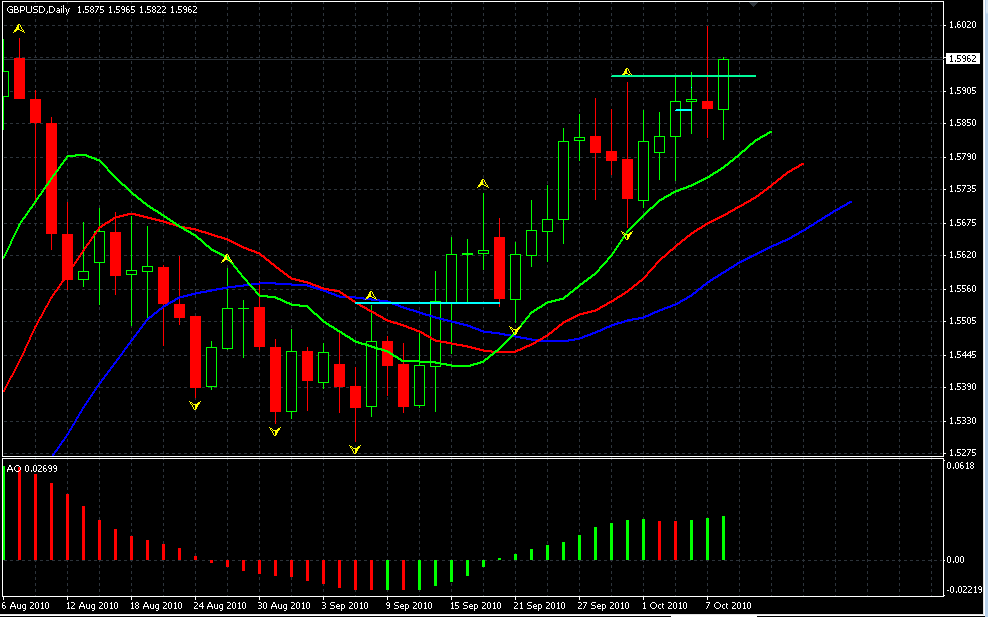Multichart ChartMany thanks for support.

Best reargds
Kinko

Multichart Code from Chaos Fractals
[LegacyColorValue = true];

Inputs: Strength(2);

value1=swinghigh(1,h,Strength,80);
value2=swinglow(1,l,Strength,80);

if value2>-1 then plot2(value2,"SellFractal");

ABC
Posts: 639
Joined: 16 Dec 2006
Has thanked: 120 times
Been thanked: 361 times
Contact:

### Re: Plot the Dot

Kinko,

you could add a piece of code shifting the dots by X ticks.
It doesn't really make sense to shift the high and low in the code below, but it should demonstrate the idea:

Code: Select all

``` Inputs: TicksToShift (2); Variables: oneTick (0), TickShift(0); once begin oneTick = MinMove/PriceScale; TickShift = oneTick * TicksToShift; end; //High shifted up TicksToShift ticks Plot1(High + TickShift, "High"); //Low shifted down TicksToShift ticks Plot2(Low - TickShift, "Low"); ```
Regards,
ABCKinko Hyo
Posts: 45
Joined: 10 Jan 2012
Has thanked: 1 time
Been thanked: 1 time

### Re: Plot the Dot

Hello ABC,

wow, thats great. Thank you very much.

Can you tell me, how i can delate the other dots?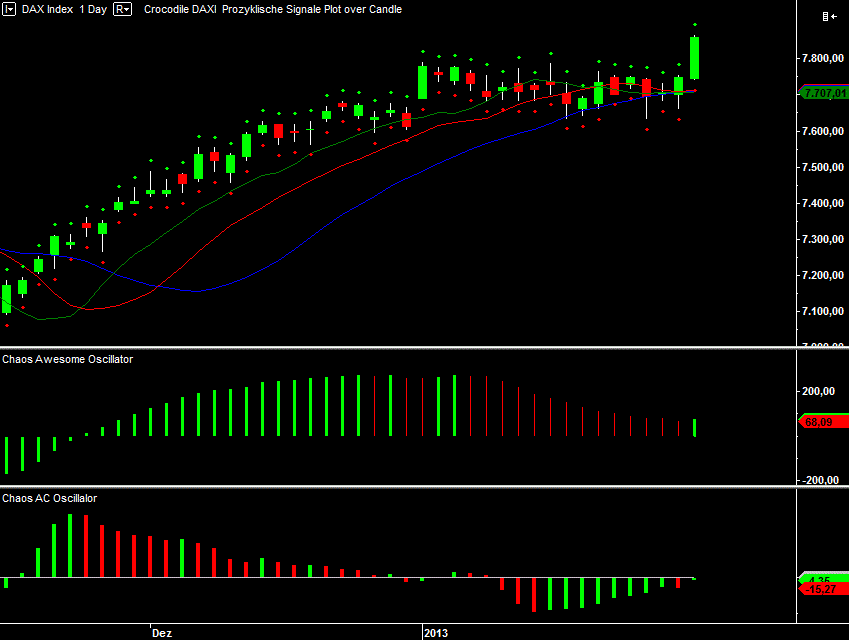Best reards
Kinko

ABC
Posts: 639
Joined: 16 Dec 2006
Has thanked: 120 times
Been thanked: 361 times
Contact:

### Re: Plot the Dot

What other dots? Which should be deleted, which should stay?
The study was only to demonstrate how you can do the shifting in general. If want it for your original study, you would just need to add the shifting part there.

Regards,
ABCKinko Hyo
Posts: 45
Joined: 10 Jan 2012
Has thanked: 1 time
Been thanked: 1 time

### Re: Plot the Dot

Hello ABC,

in the MT Chart is only one dot, please look.If i chance the formula from

to

then the dot will create over the correct candle, but the next candles are become the dot too. I will delate this dots.

The Shift Functions is very fine, thx a lot.

Best regards
KinkoKinko Hyo
Posts: 45
Joined: 10 Jan 2012
Has thanked: 1 time
Been thanked: 1 time

### Re: Plot the Dot

Hello,

i have another problem, when i plot the Indicator to the DOW Jones, i must shift to 2000, to see a difference between the dot and the high of the candle. It looks very fine like this:But when i take a stock under 10 €/\$, then the Chart ist not correkt like this.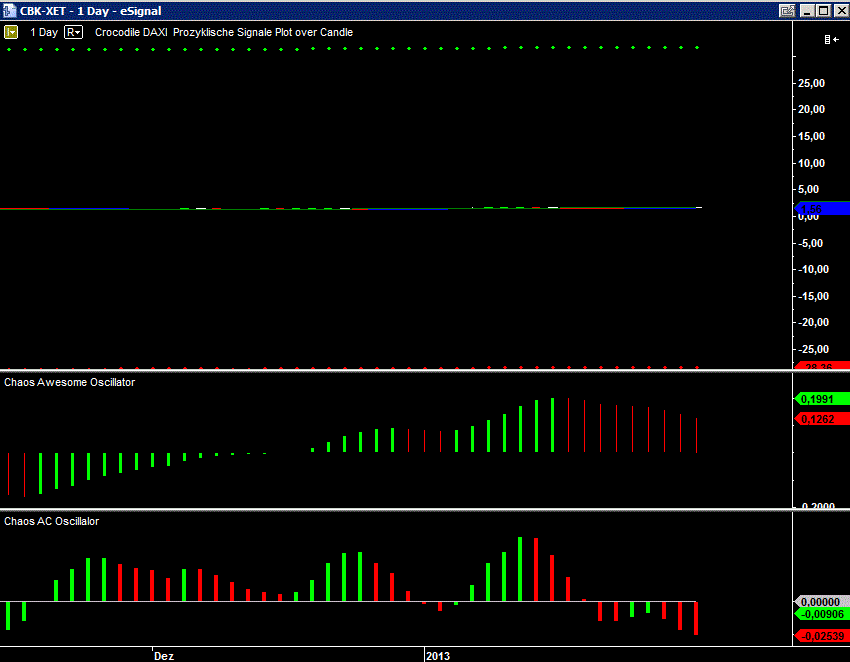Best regards
Kinko

Henry MultiСharts
Posts: 9165
Joined: 25 Aug 2011
Has thanked: 1264 times
Been thanked: 2944 times

### Re: Plot the Dot

i have another problem, when i plot the Indicator to the DOW Jones, i must shift to 2000, to see a difference between the dot and the high of the candle. It looks very fine like this:
Hello Kinko,

What is the instrument scaling in Format->Instrument->Scaling tab?
What is the study scaling in Format->Indicator->Format->Scaling tab?
What code you are using to plot the dots?Kinko Hyo
Posts: 45
Joined: 10 Jan 2012
Has thanked: 1 time
Been thanked: 1 time

### Re: Plot the Dot

Hello Henry,

The Instrument is in linear.
The Indicator ist the same as Instrument.

The Code

Code: Select all

`Plot2(Low - TickShift, "Low");`
and i try this

Code: Select all

`Plot2(Low - PanSize.Inch, "Low");}`
and this one is the best

Code: Select all

`plot2(value2-.30*truerange,"Low");`
When i try to set the Instrument to log scale, then the Multichart Software shut down.

Best regards
Kinko

Henry MultiСharts
Posts: 9165
Joined: 25 Aug 2011
Has thanked: 1264 times
Been thanked: 2944 times

### Re: Plot the Dot

Kinko Hyo, please provide screenshots of the requested Scaling tabs and the complete code that will allow replicating this behavior.Kinko Hyo
Posts: 45
Joined: 10 Jan 2012
Has thanked: 1 time
Been thanked: 1 time

### Re: Plot the Dot

Hello Henry,

here is the code:

Code: Select all

```[LegacyColorValue = true] Inputs: Strength(2), TicksToShift (2); Variables: oneTick (0), TickShift(0); value1=swinghigh(1,h,Strength,80); value2=swinglow(1,l,Strength,80); if value1>-1 then plot1(value1,"BuyFractal"); if value2>-1 then plot2(value2,"SellFractal"); once begin oneTick = MinMove/PriceScale; TickShift = oneTick * TicksToShift; end; //High shifted up TicksToShift ticks Plot1(High + TickShift, "High"); //Low shifted down TicksToShift ticks Plot2(Low - TickShift, "Low");```
In the DOW Jones Chart (\$INDU) it seems that the dots are to near by the candle.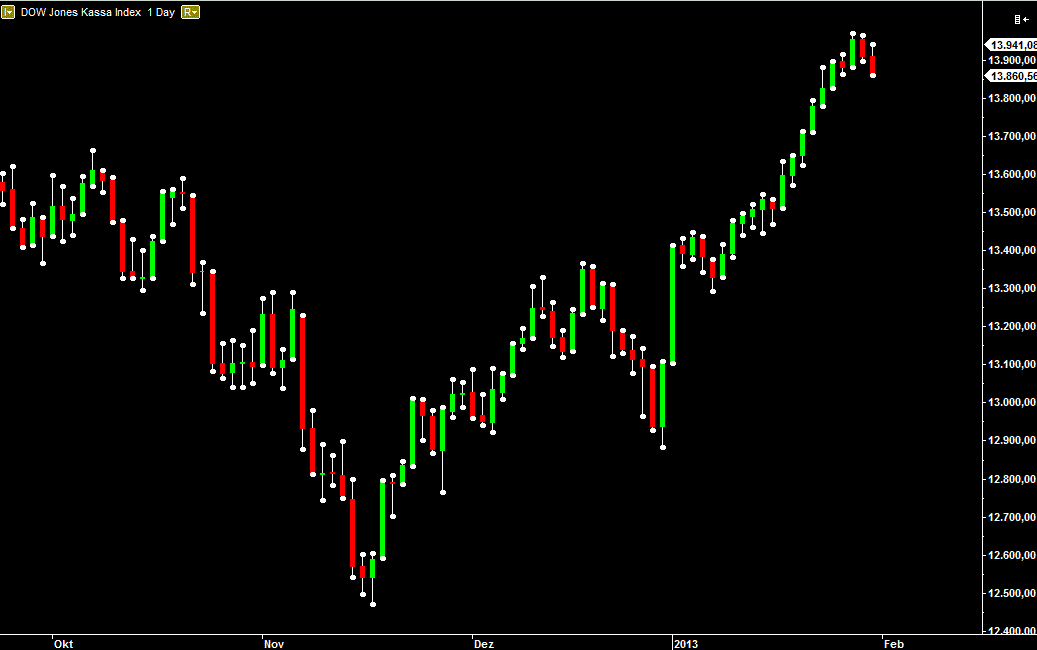In the CBK-XET Chart it looks good.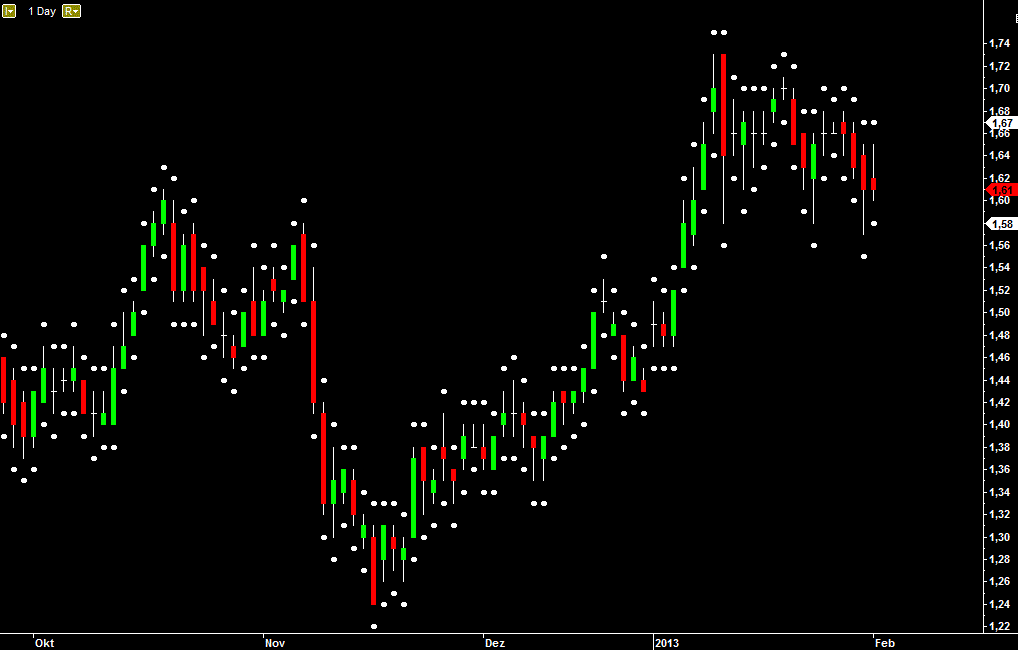Here is The Scale from the Chart: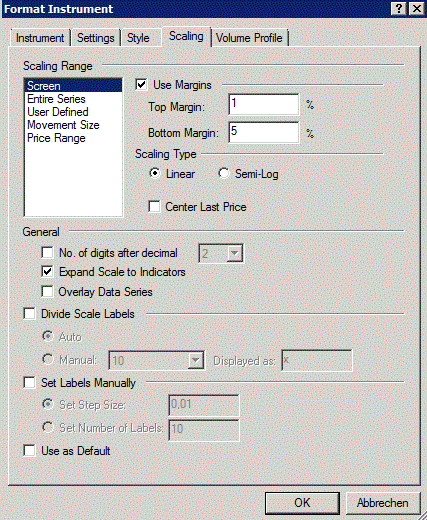And that is the scale from the indicator:When i set the tickstohift to 2000 like this: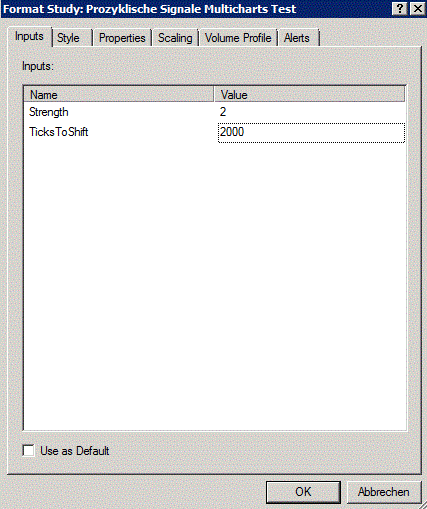Then the DOW Jones looks good.And the CBK-Chart looks terrible.So i look for an formula, where the Differenz between the high and the dot und between the low and the dot is still the same.

Best regards
Kinko

Henry MultiСharts
Posts: 9165
Joined: 25 Aug 2011
Has thanked: 1264 times
Been thanked: 2944 times

### Re: Plot the Dot

Kinko Hyo, the same TicksToShift input cannot behave the same way on these instruments as the price scale for these instruments is thousand times different. You need to set the inputs respectively to the price scales of the instruments.

Alternatively you can use the code below that will multiply the input value depending on the amount of digits in the price of the instrument. In this case the same input will do approximately the same TicksToShift for instruments with different prices:

Code: Select all

```[LegacyColorValue = true] Inputs: Strength(2), TicksToShift (2); Variables: oneTick (0), TickShift(0), Exponent(0); value1=swinghigh(1,h,Strength,80); value2=swinglow(1,l,Strength,80); if value1>-1 then plot1(value1,"BuyFractal"); if value2>-1 then plot2(value2,"SellFractal"); once begin oneTick = MinMove/PriceScale; TickShift= oneTick * TicksToShift; Exponent = 0; repeat Exponent = Exponent + 1; value3 = Power(10, Exponent); until (c > value3); If Exponent=2 then TickShift = TickShift*3; If Exponent=3 then TickShift = TickShift*30; If Exponent=4 then TickShift = TickShift*300; If Exponent=5 then TickShift = TickShift*3000; end; //High shifted up TicksToShift ticks Plot1(High + TickShift, "High"); //Low shifted down TicksToShift ticks Plot2(Low - TickShift, "Low"); //print(symbolname," ", oneTick, " ", TickShift, " ", pricescale, " ",MinMove, " ", PriceScale); ```
Attachments
Plot-the-Dot.pngKinko Hyo
Posts: 45
Joined: 10 Jan 2012
Has thanked: 1 time
Been thanked: 1 time

### Re: Plot the Dot

Hello Henry,

that looks great, many thanks.

Best regards
KinkoKinko Hyo
Posts: 45
Joined: 10 Jan 2012
Has thanked: 1 time
Been thanked: 1 time

### Re: Plot the Dot

Hello Henry,

can you please take the \$INDU and interval 1 Day and then interval 1 Minute for the \$INDU too? Can you see it?

Best regards
Kinko

Henry MultiСharts
Posts: 9165
Joined: 25 Aug 2011
Has thanked: 1264 times
Been thanked: 2944 times

### Re: Plot the Dot

Hello Henry,

can you please take the \$INDU and interval 1 Day and then interval 1 Minute for the \$INDU too? Can you see it?

Best regards
Kinko
Please be more precise in your description. What exactly are you referring to?Kinko Hyo
Posts: 45
Joined: 10 Jan 2012
Has thanked: 1 time
Been thanked: 1 time

### Re: Plot the Dot

Hello Henry,

i try it. Please look at he 5 minutes chart.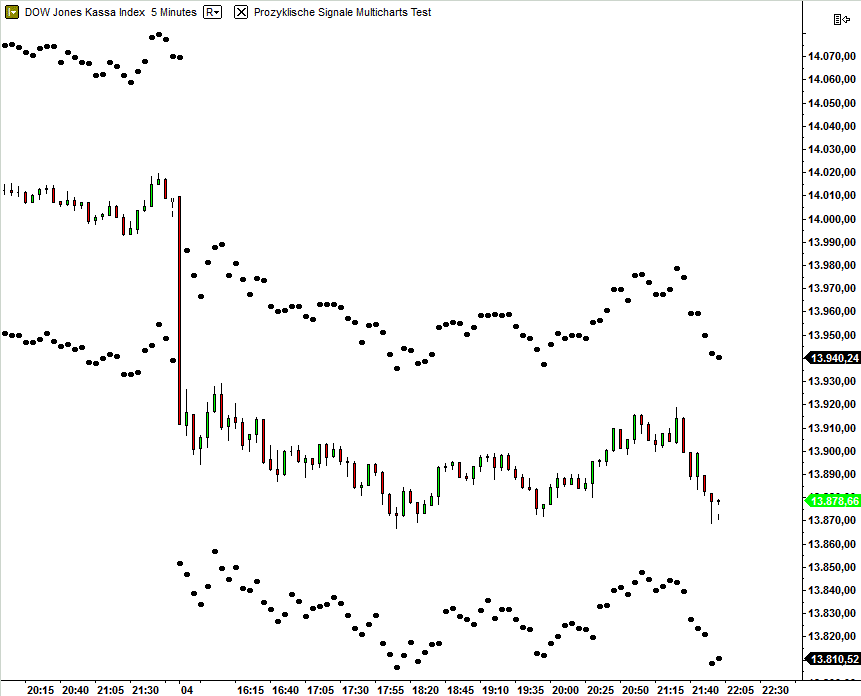And now the daily chart.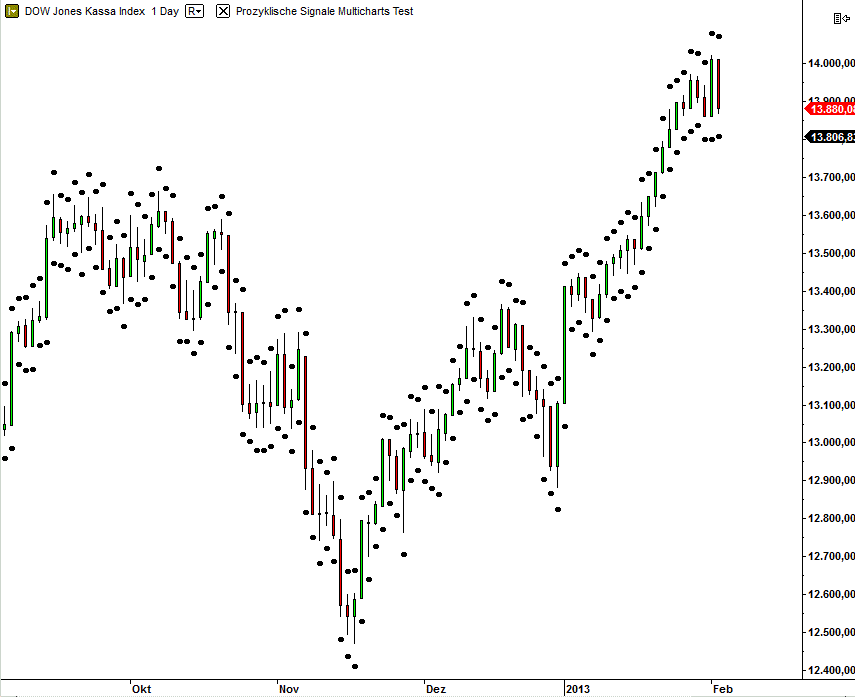And the formula:

Code: Select all

```[LegacyColorValue = true] Inputs: Strength(2), TicksToShift (2); Variables: oneTick (0), TickShift(0), Exponent(0); value1=swinghigh(1,h,Strength,80); value2=swinglow(1,l,Strength,80); {if value1>-1 then plot1(value1,"BuyFractal"); if value2>-1 then plot2(value2,"SellFractal");} once begin oneTick = MinMove/PriceScale; TickShift= oneTick * TicksToShift; Exponent = 0; repeat Exponent = Exponent + 1; value3 = Power(10, Exponent); until (c > value3); If Exponent=2 then TickShift = TickShift*3; If Exponent=3 then TickShift = TickShift*30; If Exponent=4 then TickShift = TickShift*300; If Exponent=5 then TickShift = TickShift*3000; end; //High shifted up TicksToShift ticks Plot1(High + TickShift, "High"); //Low shifted down TicksToShift ticks Plot2(Low - TickShift, "Low"); //print(symbolname," ", oneTick, " ", TickShift, " ", pricescale, " ",MinMove, " ", PriceScale);```
Best regards and thanks for your help.
KinkoSP
Posts: 455
Joined: 06 Feb 2006
Has thanked: 36 times
Been thanked: 283 times

### Re: Plot the Dot  [SOLVED]

You could adjust the shift as part of a AvgTrueRange instead of a fixed amount.

Code: Select all

``` Inputs: Strength(2), TicksToShift (5); Variables: TickShift(0); if barstatus (1) = 2 then begin TickShift = TicksToShift*reciprocal(10) * AvgTrueRange( 50 ) ; condition1 = PivotHighVSBar( 1, High, Strength, Strength, Strength+ 1 ) <> -1 ; if condition1 then Plot1[Strength]( High[Strength] + TickShift , "SellFractal" ) else NoPlot( 1 ) ; condition2 = PivotLowVSBar( 1, Low, Strength, Strength, Strength+ 1 ) <> -1 ; if condition2 then Plot2[Strength]( Low[Strength] - TickShift , "BuyFractal" ) else NoPlot( 2 ) ; end; ```Kinko Hyo
Posts: 45
Joined: 10 Jan 2012
Has thanked: 1 time
Been thanked: 1 time

### Re: Plot the Dot

Hello SP,

thank you very much, that´s fine.

Best regards
Kinko